# Volume of a Rectangular Prism with Fractional Edge Lengths Online Quiz

#### Le Guide Définitif du Développement Web HTML.

76 Lectures 3.5 hours

#### SAP ERP : Gestion magasin WM (débutant et avancé)

66 Lectures 4.5 hours

#### Initiation SAP ERP débutant - Les Bases

12 Lectures 1 hours

Following quiz provides Multiple Choice Questions (MCQs) related to Volume of a Rectangular Prism with Fractional Edge Lengths. You will have to read all the given answers and click over the correct answer. If you are not sure about the answer then you can check the answer using Show Answer button. You can use Next Quiz button to check new set of questions in the quiz.Q 1 - Find the volume of following rectangular prism with fractional edge lengths. Each prism unit is measured in cm (not to scale)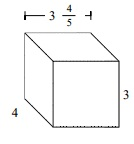### Explanation

Step 1:

The given prism has length = $3 \frac{4}{5}$; width = 4; height = 3

Step 2:

The volume of prism V = l × w × h

= $3 \frac{4}{5}$ × 4 × 3

= $45 \frac{3}{5}$ cubic units.

Q 2 - Find the volume of following rectangular prism with fractional edge lengths. Each prism unit is measured in cm (not to scale)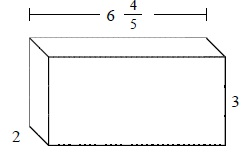### Explanation

Step 1:

The given prism has length = $6 \frac{4}{5}$; width = 2; height = 3

Step 2:

The volume of prism V = l × w × h

= $6 \frac{4}{5}$ × 2 × 3

= $40 \frac{4}{5}$ cubic units.

Q 3 - Find the volume of following rectangular prism with fractional edge lengths. Each prism unit is measured in cm (not to scale)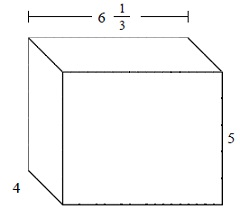### Explanation

Step 1:

The given prism has length = $6 \frac{1}{3}$; width = 4; height = 5

Step 2:

The volume of prism V = l × w × h

= $6 \frac{1}{3}$ × 4 × 5

= $126 \frac{2}{3}$ cubic units.

Q 4 - Find the volume of following rectangular prism with fractional edge lengths. Each prism unit is measured in cm (not to scale)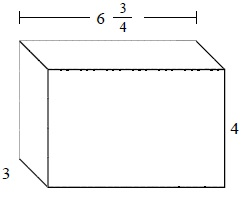### Explanation

Step 1:

The given prism has length = $6 \frac{3}{4}$; width = 3; height = 4

Step 2:

The volume of prism V = l × w × h

= $6 \frac{3}{4}$ × 3 × 4

= 81 cubic units.

Q 5 - Find the volume of following rectangular prism with fractional edge lengths. Each prism unit is measured in cm (not to scale)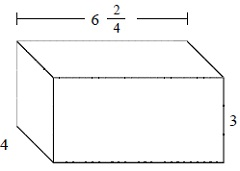### Explanation

Step 1:

The given prism has length = $6 \frac{2}{4}$; width = 4; height = 3

Step 2:

The volume of prism V = l × w × h

= $6 \frac{2}{4}$ × 4 × 3

= 78 cubic units.

Q 6 - Find the volume of following rectangular prism with fractional edge lengths. Each prism unit is measured in cm (not to scale)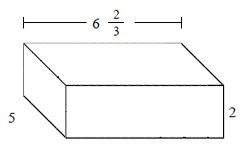### Explanation

Step 1:

The given prism has length = $6 \frac{2}{3}$; width = 5; height = 2

Step 2:

The volume of prism V = l × w × h

= $6 \frac{2}{3}$ × 5 × 2

= $66 \frac{2}{3}$ cubic units.

Q 7 - Find the volume of following rectangular prism with fractional edge lengths. Each prism unit is measured in cm (not to scale)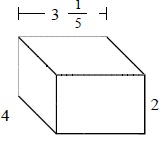### Explanation

Step 1:

The given prism has length = $3 \frac{1}{5}$; width = 4; height = 2

Step 2:

The volume of prism V = l × w × h

= $3 \frac{1}{5}$ × 4 × 2

= $25 \frac{3}{5}$ cubic units.

Q 8 - Find the volume of following rectangular prism with fractional edge lengths. Each prism unit is measured in cm (not to scale)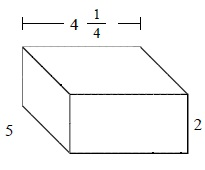### Explanation

Step 1:

The given prism has length = $4 \frac{1}{4}$; width = 5; height = 2

Step 2:

The volume of prism V = l × w × h

= $4 \frac{1}{4}$ × 5 × 2

= $42 \frac{1}{2}$ cubic units.

Q 9 - Find the volume of following rectangular prism with fractional edge lengths. Each prism unit is measured in cm (not to scale)### Explanation

Step 1:

The given prism has length = $6 \frac{2}{3}$; width = 4; height = 3

Step 2:

The volume of prism V = l × w × h

= $6 \frac{2}{3}$ × 4 × 3

= 80 cubic units.

Q 10 - Find the volume of following rectangular prism with fractional edge lengths. Each prism unit is measured in cm (not to scale)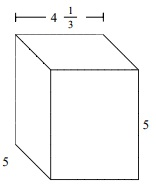### Explanation

Step 1:

The given prism has length = $4 \frac{1}{3}$; width = 5; height = 5

Step 2:

The volume of prism V = l × w × h

= $4 \frac{1}{3}$ × 5 × 5

= $108 \frac{1}{3}$ cubic units.

volume_of_rectangular_prism_with_fractional_edge_lengths.htm